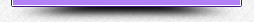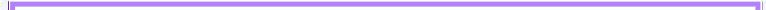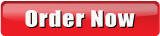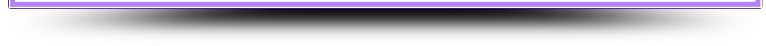1.877.974.8398

Toronto: 416.222.5225         Vancouver: 604.757.5246
support@essayexperts.ca## Essay Writing

We've successfully helped 50,000+ university students in Canada with our premium custom essays.

## Thesis & Dissertation

Our Thesis and Dissertation division has helped over 15,000 graduate students whether it's one chapter or all five. Find out more.

## How We WorkHow to Order

Current Service Hours

Mon-Wed:  10 am - 11 pm
Thu-Fri:  10 am - 11 pm
Sat-Sun:    1 pm - 9 pm
------------------

*Walk-in Visit by Appointment Only

Essay Experts Local
Expert Extras# Statistics Subject Services ListThe following is a service list of the vast variety of statistical areas we cover. The easiest way to handle any request is to send us your information, question sheet or assignment page. We’ll communicate with you to give you a quote and can explain the process to you:

1. Qualitative and Quantitative Data Types
(Binomial, nominal, ordinal, ratio, discrete and continuous/scale data)
2. Data Cleaning and Sampling
(ungrouped and grouped data organization)
3. Descriptive Statistics and Analysis
(measures of frequency – count, percent and frequency, measures of central tendency- mean, median and the mode, measures of variation- range, standard deviation, and variance, measures of position- IQR, percentile ranks, and quartile ranks)
4. Graphical and Visual Data Presentation
(bara charts, scatter plots, pie charts, histograms, boxplots, heat maps, ogives, and polygons)
5. Probabilities
(simple, conditional, marginal union, intersection, additional and multiplication, independent and mutually exclusive event probabilities)
6. Probability Distributions
(Uniform- to model multiple events, Binomial- to model binary data, and Poisson distribution- to model count data)
7. Sampling and sampling distributions
(sampling methods, the central limit theorem, margin, and Standard errors)
8. Sample Size Estimation and Power Analysis
(effect size, power analysis)
9. Confidence Intervals
(population parameters- mean and variance, point estimates- proportions)
10. Tests of Statistical Significance
(hypothesis testing, level of significance, one and two-tailed tests, parametric and non-parametric tests, critical value and p-value approach, Type I and Type II errors, effect size, observed power)
11. Test of Data Normality
(Kolmogorov-Smirnov and Shapiro-Wilk tests)
12. Hypothesis testing 1 or 2 populations
(equality of variances and Levene's F test, t-test, z-test, Welch's t-test)
13. Repeated Measures Design
(paired samples t-test, repeated measures ANOVA)
14. Tests of Association
(chi-square goodness of fit test, chi-square test of independence)
15. Correlational Analysis (Pearson’s correlations, Spearman’s Rank-order correlations, partial correlations)
16. Analysis of Variance
(One-way ANOVA, Two-way ANOVA, Sphericity, Interaction effects, Repeated measures ANOVA, ANCOVA, and MANOVA)
17. Post-Hoc-Analysis
(Tukey’s test- Studentized range distribution, Bonferroni procedures, Duncan’s Multiple range test, Dunn’s multiple comparison test, Fishers least significant difference-LSD, Holm-Bonferroni process, Newman-Keuls, Rodgers method, and Scheffe)
18. Regression Analysis
(simple linear and multiple regressions, ordinary least squares regression Logistic and multinomial regressions, ridge and lasso regressions, Multi-collinearity, coefficient of determination, testing of significance, regression equation modeling, confidence and prediction intervals, testing assumptions, and residual analysis)
19. Non-Parametric Analysis of Relationship
(Chi-square, Fisher's Exact test, Phi and Cramer's V, Lambda, Gamma, Kendall's Tau)
20. Non-parametric Hypothesis Testing
(Mann-Whitney U test, Kruskal-Wallis ANOVA by ranks, Wilcoxon Signed-Rank test, Friedman repeated measures test)
21. Factor Analysis
(exploratory and confirmatory analysis, structural equation modeling)
22. Cluster Analysis
(centroid clustering, density clustering, distribution clustering, and connectivity clustering)
23. Research Designs/Methods
(experimental design - controlled experiment vs observational study, external and internal validity, measurement reliability and validity, case study design, causal design, cohort designs, cross-sectional design, and descriptive design)
24. Causality analysis
(confounding factors, intervening variables- mediator and moderator elements, Sobel’s z-test)
25. Linear Programming
(decision tree models, objective functions, optimization- simplex, graphical method, and open solver)
26. Meta-analysis
(heterogeneity analysis, sensitivity analysis, and evaluation of publication bias)>>> Take a minute to place your order and give us all your details – you’ll be glad you did!

Essay Experts is your one stop for all your statistics and related help. Whether you have a question about your assignment or our statisticians we are available to help.